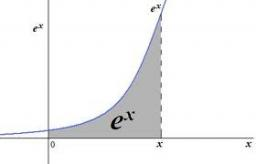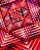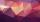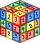# Geometric progression 2

There is geometric sequence with a1=5.7 and quotient q=-2.5. Calculate a17.

a17 =  13271346.69

### Step-by-step explanation:

${a}_{17}=5.7\cdot \left(-2.5{\right)}^{17-1}\doteq 13271346.69$Did you find an error or inaccuracy? Feel free to write us. Thank you!## Related math problems and questions:

• Geometric progressionIn geometric progression, a1 = 7, q = 5. Find the condition for n to sum first n members is sn≤217.
• Tenth memberCalculate the tenth member of geometric sequence when given: a1=1/2 and q=2
• Sequence 11What is the nth term of this sequence 1, 1/2, 1/3, 1/4, 1/5?
• Five elementThe geometric sequence is given by quotient q = 1/2 and the sum of the first six members S6 = 63. Find the fifth element a5.
• Geometric sequenceIn the geometric sequence is a4 = 20 a9 = -160. Calculate the first member a1 and quotient q.
• Meanif the mean of the set of data 5, 17, 19, 14, 15, 17, 7, 11, 16, 19, 5, 5, 10, 8, 13, 14, 4, 2, 17, 11, x is -91.74, what is the value of x?
• Q of GPCalculate quotient of geometric progression if a1=5 a a1+a2=12.
• Geometric sequence 5About members of geometric sequence we know: ? ? Calculate a1 (first member) and q (common ratio or q-coefficient)
• SequenceCalculate what member of the sequence specified by (5n-2+15) has value 20.
• Five membersWrite first 5 members geometric sequence and determine whether it is increasing or decreasing: a1 = 3 q = -2
• QuotientDetermine the quotient and the second member of the geometric progression where a3=10, a1+a2=-1,6 a1-a2=2,4.
• GP membersThe geometric sequence has 10 members. The last two members are 2 and -1. Which member is -1/16?
• SequenceFind the common ratio of the sequence -3, -1.5, -0.75, -0.375, -0.1875. Ratio write as decimal number rounded to tenth.
• Geometric sequence 3In geometric sequence is a8 = 312500; a11= 39062500; sn=1953124. Calculate the first item a1, quotient q, and n - number of members by their sum s_n.
• SequenceWrite the first 6 members of this sequence: a1 = 5 a2 = 7 an+2 = an+1 +2 an
• Sequence 2Write the first 5 members of an arithmetic sequence a11=-14, d=-1
• Geometric sequence 4It is given geometric sequence a3 = 7 and a12 = 3. Calculate s23 (= sum of the first 23 members of the sequence).